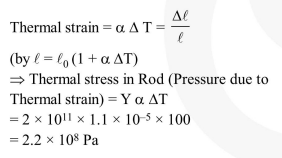Deepak Scored 45->99%ile with Bounce Back Crack Course. You can do it too!

# The pressure that has to be applied to the ends of a steel wire of length

Question:

The pressure that has to be applied to the ends of a steel wire of length $10 \mathrm{~cm}$ to keep its length constant when its temperature is raised by $100^{\circ} \mathrm{C}$ is :

(For steel Young's modulus is $2 \times 10^{11} \mathrm{~N} \mathrm{~m}^{-2}$ and coefficient of thermal expansion is $1.1 \times 10^{-5} \mathrm{~K}^{-1}$ )

1. $2.2 \times 10^{7} \mathrm{~Pa}$

2. $2.2 \times 10^{6} \mathrm{~Pa}$

3. $2.2 \times 10^{8} \mathrm{~Pa}$

4. $2.2 \times 10^{9} \mathrm{~Pa}$

Correct Option: , 3

Solution: